# simplicial approximation

The simplicial complex is an algebraic foundation of broader area of mathematics that carries combinatorial information based on the topology. In particular, the well-developed methods of construction and their calculation are significant to apply in real-life data science.

To analyze the topology of data by means of some well known construction such as Čech’s, we want an arbitrary map between spaces under some reasonable constraints to well behave around some representing point of data, in a sense that the map does not disregard too much of the combinatorics.

Let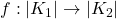be a continuous map between realizations of simplicial complexes and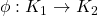a simplicial map.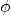is said to be a simplicial approximation of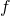if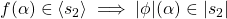(equivalently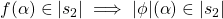). At the first sight, this is quite a formal description that the objective is not apparent. When exists, the key properties of such approximationtois that it should suffice:

• homotopic to,
• bothandsend each point into the same simplex,
• the difference is small enough to respect subcomplex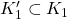over whichandcoincides.

Keeping these in mind, consider the equivalent statement:

•,
•.

The later statement automatically implies the former (the former is under more strict condition). From the former to later, we should not mistakenly appeal to the simple topological fact that for an open set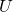and closed set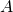with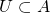,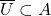; because it is not true that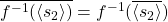in general (only whenis local homeomorphism) despite that the former statement can be restated as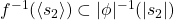.

For the rigorous proof of the equivalence, we note that realized simplices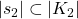always are compact metric space, which is sequentially compact (i.e. every sequence has convergent subsequence), which is complete (i.e. every Cauchy sequence converges within the set). Assuming the former statement implies negation of later, in which we have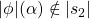for some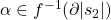. Choose a convergent sequence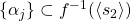such that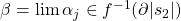and an open neighborhoodof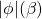with property that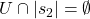. Then, by continuity of, for infinitely many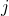,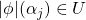. Therefore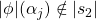for some (infinitely many), contradiction.

To see the approximation as an “operation” to a continuous function between simplicial complexes, there are some notable properties:

• simplicial approximation is idempotent (simplicial approximation to a given simplicial mapis equivalent to),
• simplicial approximation is compatible with composition (of maps);

finally, we have the notable simplicial approximation theorem:

Theorem. a map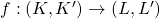between simplicial pairs admits simplicial approximation. In particular if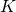is finite, the approximation can be taken over iterated (barycentric) subdivision of.

Applying the previous theorem, we have some fundamental results in the homotopy theory regarding the homotopy group of a hypersphere. The proof is from Hatcher’s and it uses the key fact that the simplicial map sends a simplex to a simplex of dimension equal to or less than (called degenerate simplex) the original.

Proposition.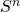is (n-1)-connected for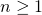.

Proof. For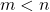, it is sufficient to show that any map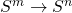is null-homotopic. Let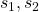be (m+1) and (n+1) simplices, respectively. We have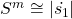and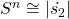and any map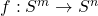admits simplicial approximation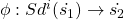. Because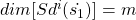,maps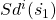to m-skeleton of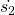. Hencefactors into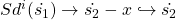for some point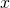, which is null-homotopic since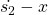is contractible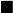Proposition. For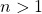, any map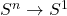is null-homotopic.

Proof. By the previous theorem,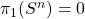. Since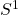is path-connected and locally path-connected, a mapfactors through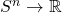by the lifting theorem